# (Older Version) MathML Browser Test (Presentation Markup)

This is an XHTML 1.1 plus MathML 2.0 document.
Click on a formula/equation to see the source code that generated it.
Try viewing the newer HTML5 version of this document.

Formula Image of TeX rendering
(MiKTeX 2.9)
Image of MathML rendering
(Firefox 4.0 with STIX Fonts)
MathML rendering
(by this browser)
Axiom of
power set$\forall A\exists P\forall B\phantom{\rule{thinmathspace}{0ex}}\left[B\in P⟺\forall C\phantom{\rule{thinmathspace}{0ex}}\left(C\in B⇒C\in A\right)\right]$
De Morgan's law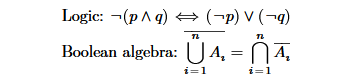Formula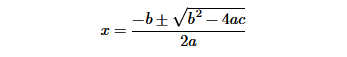$x=\frac{-b±\sqrt{{b}^{2}-4ac}}{2a}$
Binomial
Coefficient$C\left(n,k\right)={C}_{k}^{n}={}_{n}C_{k}=\left(\genfrac{}{}{0}{}{n}{k}\right)=\frac{n!}{k!\left(n-k\right)!}$
Sophomore's
dream${\int }_{0}^{1}{x}^{x}dx=\sum _{n=1}^{\infty }{\left(-1\right)}^{n+1}{n}^{-n}$
Divergence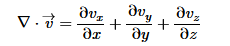$\nabla ·\stackrel{\to }{v}=\frac{\partial {v}_{x}}{\partial x}+\frac{\partial {v}_{y}}{\partial y}+\frac{\partial {v}_{z}}{\partial z}$
Complex
number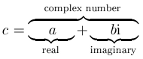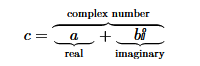$c=\stackrel{\text{complex number}}{\overbrace{\underset{\text{real}}{\underbrace{\phantom{\rule{1.2em}{0ex}}a\phantom{\rule{1.2em}{0ex}}}}+\underset{\text{imaginary}}{\underbrace{\phantom{\rule{1em}{0ex}}bi\phantom{\rule{1em}{0ex}}}}}}$
Moore
determinant$M=\left[\begin{array}{cccc}{\alpha }_{1}& {\alpha }_{1}^{q}& \dots & {\alpha }_{1}^{{q}^{n-1}}\\ {\alpha }_{2}& {\alpha }_{2}^{q}& \dots & {\alpha }_{2}^{{q}^{n-1}}\\ ⋮& ⋮& \ddots & ⋮\\ {\alpha }_{m}& {\alpha }_{m}^{q}& \dots & {\alpha }_{m}^{{q}^{n-1}}\end{array}\right]$
Sphere
volume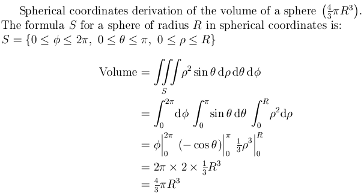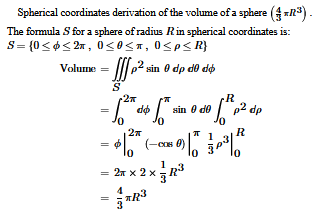Spherical coordinates derivation of the volume of a sphere $\left(\frac{4}{3}\pi {R}^{3}\right)$ .
The formula $S$ for a sphere of radius $R$ in spherical coordinates is:
$S=\left\{0\le \varphi \le 2\pi ,0\le \theta \le \pi ,0\le \rho \le R\right\}$
$\begin{array}{rl}\text{Volume}& =\underset{S}{\iiint }{\rho }^{2}sin\theta d\rho d\theta d\varphi \\ & ={\int }_{0}^{2\pi }d\varphi {\int }_{0}^{\pi }sin\theta d\theta {\int }_{0}^{R}{\rho }^{2}d\rho \\ & =\phantom{\rule{mediummathspace}{0ex}}\varphi \phantom{\rule{verythinmathspace}{0ex}}{|}_{\phantom{\rule{verythinmathspace}{0ex}}0}^{\phantom{\rule{verythinmathspace}{0ex}}2\pi }\left(-\mathrm{cos}\theta \right)\phantom{\rule{verythinmathspace}{0ex}}{|}_{\phantom{\rule{verythinmathspace}{0ex}}0}^{\phantom{\rule{verythinmathspace}{0ex}}\pi }\phantom{\rule{veryverythickmathspace}{0ex}}\frac{1}{3}{\rho }^{3}\phantom{\rule{verythinmathspace}{0ex}}{|}_{\phantom{\rule{verythinmathspace}{0ex}}0}^{\phantom{\rule{verythinmathspace}{0ex}}R}\\ & =\phantom{\rule{mediummathspace}{0ex}}2\pi ×2×\frac{1}{3}{R}^{3}\\ & =\phantom{\rule{mediummathspace}{0ex}}\frac{4}{3}\pi {R}^{3}\end{array}$
Schwinger-Dyson
equation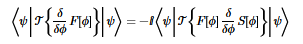$⟨\psi \phantom{\rule{thinmathspace}{0ex}}\left|\phantom{\rule{thinmathspace}{0ex}}𝒯\left\{\frac{\delta }{\delta \varphi }F\left[\varphi \right]\right\}\right|\phantom{\rule{thinmathspace}{0ex}}\psi ⟩=-i⟨\psi \phantom{\rule{thinmathspace}{0ex}}\left|\phantom{\rule{thinmathspace}{0ex}}𝒯\left\{F\left[\varphi \right]\frac{\delta }{\delta \varphi }S\left[\varphi \right]\right\}\right|\phantom{\rule{thinmathspace}{0ex}}\psi ⟩$
Differentiable
Manifold
(tangent vector)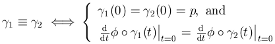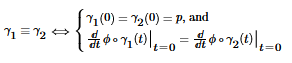${\gamma }_{1}\equiv {\gamma }_{2}⟺\left\{\begin{array}{l}{\gamma }_{1}\left(0\right)={\gamma }_{2}\left(0\right)=p\text{, and}\\ {\frac{d}{dt}\varphi \circ {\gamma }_{1}\left(t\right)\phantom{\rule{verythinmathspace}{0ex}}|}_{\phantom{\rule{verythinmathspace}{0ex}}t=0}={\frac{d}{dt}\varphi \circ {\gamma }_{2}\left(t\right)\phantom{\rule{verythinmathspace}{0ex}}|}_{\phantom{\rule{verythinmathspace}{0ex}}t=0}\end{array}\right\$
Cichoń's
Diagram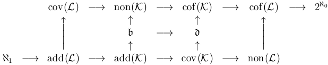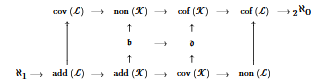$\begin{array}{ccccccccccc}\multicolumn{2}{c}{}& cov\left(ℒ\right)& ⟶& non\left(𝒦\right)& ⟶& cof\left(𝒦\right)& ⟶& cof\left(ℒ\right)& ⟶& {2}^{{\aleph }_{0}}\\ \multicolumn{2}{c}{}& ↑& & ↑& & ↑& & ↑& \multicolumn{2}{c}{}\\ \multicolumn{4}{c}{}& 𝔟& ⟶& 𝔡& \multicolumn{5}{c}{}\\ \multicolumn{4}{c}{}& ↑& & ↑& \multicolumn{5}{c}{}\\ {\aleph }_{1}& ⟶& add\left(ℒ\right)& ⟶& add\left(𝒦\right)& ⟶& cov\left(𝒦\right)& ⟶& non\left(ℒ\right)& \multicolumn{2}{c}{}\end{array}$
multiscripts
&
greek alphabet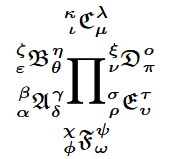$\underset{{}_{\varphi }{}^{\chi }𝔉_{\omega }^{\psi }}{\overset{{}_{\iota }{}^{\kappa }ℭ_{\mu }^{\lambda }}{{}_{{}_{\alpha }{}^{\beta }𝔄_{\delta }^{\gamma }}{}^{{}_{\epsilon }{}^{\zeta }𝔅_{\theta }^{\eta }}\prod _{{}_{\rho }{}^{\sigma }𝔈_{\upsilon }^{\tau }}^{{}_{\nu }{}^{\xi }𝔇_{\pi }^{ο}}}}$
nested roots$\frac{\sqrt{1+\sqrt{2+\sqrt{3+\sqrt{4+\sqrt{5+\sqrt{6+\sqrt{7+\sqrt{A}}}}}}}}}{{e}^{\pi }}={x}^{‴}$
nested
matrices$\left(\begin{array}{cc}\left(\begin{array}{cccc}{a}_{1}& {a}_{2}& {a}_{3}& {a}_{4}\\ {a}_{5}& {a}_{6}& {a}_{7}& {a}_{8}\end{array}\right)& \left(\begin{array}{c}{b}_{1}\\ {b}_{2}\\ \phantom{\rule{0ex}{1.4em}}{b}_{3}\\ {b}_{4}\end{array}\right)\\ \begin{array}{cc} 0 & \left(\begin{array}{cc}{c}_{1}& {c}_{2}\\ {c}_{3}& {c}_{4}\end{array}\right)\end{array}& \end{array}\right)$
font sizes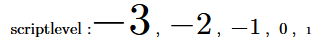$\text{scriptlevel :}-3,-2,-1,0,1$

## NOTES:

I hope this site can be used as a learning aid (tutorial by example) for mathematics in TeX/LaTeX and in coding MathML.
A small sample of many different types of mathematical expressions and equations is shown.
All the examples are complete with the source code available. (Just click on the equation/formula.)

This web page (XHTML document) was validated as:

### Lessons Learned Working on MathML with STIX Fonts on Firefox:

When using an `mtable`, the table cell (`mtd`) default vertical padding produces excessive spacing. Setting the top and bottom padding to zero "`0`" fixes this.

When using the `mfenced` tag, the "fences" have no spacing around them.
When using the vertical bar "|" (`&vert;`) as a fence, adding a little spacing around it improves the readability of the result.

Firebug is an add-on to the Firefox browser. It is a great development tool that works well with MathML.